# Decagon facts for kids

Kids Encyclopedia Facts

A decagon is a shape with 10 sides and 10 corners.

## Regular decagon

All sides of a regular decagon are the same length. Each corner is 144°. All corners added together equal 1440°.

### Area

The amount of space a regular decagon takes up is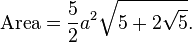$\text{Area} = \frac{5}{2} a^2\sqrt{5+2\sqrt{5}}.$

a is the length of one of its sides.

An alternative formula is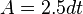$A = 2.5dt$ where d is the distance between parallel sides, or the height when the decagon stands on one side as base.
By simple trigonometry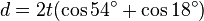$d = 2t(\cos{54^\circ} + \cos{18^\circ})$.

## Sides

The side of a regular decagon inscribed in a unit circle is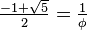$\tfrac{-1+\sqrt{5}}{2}=\tfrac{1}{\phi}$, where ϕ is the golden ratio,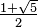$\tfrac{1+\sqrt{5}}{2}$.

## Dissection of regular decagon

Coxeter states that every parallel-sided 2m-gon can be divided into m(m-1)/2 rhombs. For the regular decagon, m=5, and it can be divided into 10 rhombs, with examples shown below. This decomposition can be seen as 10 of 80 faces in a Petrie polygon projection plane of the 5-cube. A dissection is based on 10 of 30 faces of the rhombic triacontahedron. The list A006245 defines the number of solutions as 62, with 2 orientations for the first symmetric form, and 10 orientations for the other 6.

## Skew decagon

3 regular skew zig-zag decagons
{5}#{ } {5/2}#{ } {5/3}#{ }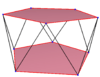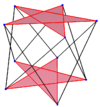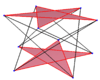A regular skew decagon is seen as zig-zagging edges of a pentagonal antiprism, a pentagrammic antiprism, and a pentagrammic crossed-antiprism.

A skew decagon is a skew polygon with 10 vertices and edges but not existing on the same plane. The interior of such an decagon is not generally defined. A skew zig-zag decagon has vertices alternating between two parallel planes.

A regular skew decagon is vertex-transitive with equal edge lengths. In 3-dimensions it will be a zig-zag skew decagon and can be seen in the vertices and side edges of a pentagonal antiprism, pentagrammic antiprism, and pentagrammic crossed-antiprism with the same D5d, [2+,10] symmetry, order 20.

These can also be seen in these 4 convex polyhedra with icosahedral symmetry. The polygons on the perimeter of these projections are regular skew decagons.

## Related pages

• Decagonal number and centered decagonal number, figurate numbers modeled on the decagon
• Decagram, a star polygon with the same vertex positions as the regular decagon

## Images for kidsDecagon Facts for Kids. Kiddle Encyclopedia.